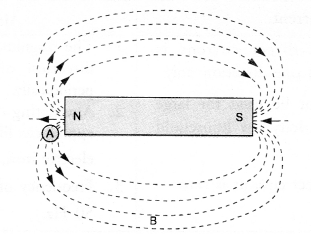## HOTS Questions for Class 10 Science Chapter 13 Magnetic Effects of Electric Current

These Solutions are part of HOTS Questions for Class 10 Science. Here we have given HOTS Questions for Class 10 Science Chapter 13 Magnetic Effects of Electric Current

Question 1.
One end of a bar magnet is brought near the south pole of a magnetic compass needle. It was noticed that the needle of the magnetic compass deflected away from the end of the bar magnet. Name the pole of the bar magnet at its end pointing towards the south pole of the magnetic compass. Justify your answer.
The pole of the bar magnet at the end pointing towards the south pole of the magnetic compass is south pole. This is because, like magnetic poles repel each other.

More Resources

Question 2.
Magnetic field lines around a bar magnet are shown in figure. A student makes a statement that magnetic field at point A is stronger than at point B. State, whether the statement is correct or incorrect. Explain.
(CBSE 2012)Statement is correct. This is because field lines are crowded in a region of strong magnetic field and field lines diverge in a region of weak magnetic field.

Question 3.
Magnetic lines of force of two pairs of magnets are shown in figure A and B. Out of these two figures, which one represents the correct pattern of field lines. Name the poles of magnets facing each other. (CBSE 2012)Figure B represents the correct pattern of magnetic field lines of a pair of magnets. Figure A does not represent the correct pattern of field lines because magnetic field lines never cross each other.Poles of magnets facing each other are north poles as the magnetic field lines emerge from a magnet at N-pole.

Question 4.
A student performs an experiment to study the magnetic effect of current around a current carrying straight conductor. He reports that

1. the direction of deflection of the north pole of a compass needle kept at a given point near the conductor remains unaffected even when the terminals of the battery sending current in the wire are interchanged.
2. for a given battery, the degree of deflection of a N-pole decreases when the compass is kept at a point farther away from the conductor. Which of the above observations of the student is incorrect and why ?
(CBSE Sample Paper Question)

1. The first statement is incorrect because the direction of the magnetic field around the conductor is reversed when the direction of the current flowing in the conductor changes. Hence, the direction of deflection of N-pole of the compass needle cannot remain unaffected when the terminals of the battery sending current in the wire or conductor are interchanged.
2. The second statement is correct because the magnetic field decreases with the increase in the distance from the conductor.

Question 5.
A current carrying conductor is placed perpendicular to the magnetic field of horse-shoe magnet. The
conductor is displaced upward. What will happen to the displacement of the conductor if

1. current in the conductor is increased,
2. a horse-shoe magnet is replaced by another stronger horse-shoe magnet and
3. the length of the conductor is increased. (Similar Text Book Question)